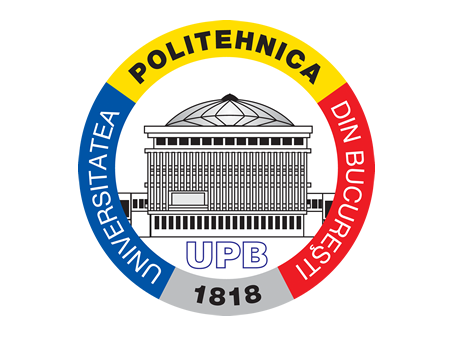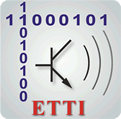Digital Signal Processing

Course in Bachelor programme (3rd year, series E)

Teachers

Teacher: Prof. Dragoş Burileanu
Teaching Assistant: As. Drd. Şerban Mihalache

Course Description

The course presents and discusses the fundamentals of digital signal processing: specific algorithms, processing techniques, VLSI architectures, and practical considerations for implementation. The aim is to understand the phenomena associated with the major DSP techniques, viewed from the system designer point of view. Examples and detailed explanations help clarify the most difficult theoretical issues.

The laboratory’s aim is practical learning of the main concepts in the digital signal processing domain. Applications include various software simulations using a high-level programming environment (Matlab).

Contents

Course

• Introduction. Comparison with analog solutions; performance and limitations in digital signal processing
• Time-domain representation of discrete signals and systems
• Frequency-domain representation of discrete signals and systems
• Analysis of discrete systems using the z-transform
• The Discrete Fourier Transform (DFT). Window functions; frequency resolution
• The Fast Fourier Transform (FFT). Practical aspects of FFT implementation and optimization techniques
• Digital filter design and analysis. IIR and FIR filters; computation complexity
• Finite-precision arithmetic in digital signal processing
• Hardware implementation of DSP systems. VLSI architectures, development systems and practical considerations of DSP algorithm implementation

Laboratory

• Presentation of the Matlab software package; examples. Generation and time-domain representation of discrete sequences
• Time-domain representation of discrete systems: convolution, impulse response, stability; the digital filtering concept
• Frequency-domain representation of discrete signals. DFT and FFT: applications, spectral analysis
• Digital filters – synthesis, analysis, implementation
• Digital filters for audio applications
• Review. Miscellaneous issues in DSP
• Laboratory assessment